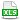HomeTroubleshootingPrecisionTree#VALUE in Tree with Utility Function

# 12.5. #VALUE in Tree with Utility Function

Applies to: PrecisionTree 5.x and newer

My tree seems okay when I'm just doing regular calculations. but when I switch to using a utility function, some of the branches' values change to #VALUE.

This depends on the tree, your utility function, and your R value, but basically one or more of your branch values leads to a forbidden mathematical operation. The attached workbook shows two simple trees with this problem. To see what's going on with each tree, you may find it helpful to open Model Settings, select the Utility Function tab, and change display to Expected Utility.

Depending on your utility function, here are some hints on what to look for.

Suppose you are using the built-in Exponential utility function,

U = 1 − exp(−\$/R)

You won't have a problem displaying Expected Values, but you may have a problem with Certainty Equivalents. For greater and greater positive branch values, this function gets closer and closer to 1. If the Expected Utility of any node displays as 1, it isn't actually 1, but the difference between the utility and 1 is too small to be represented in double precision arithmetic. Thus the utility function treats the utility of any sufficiently large branch value (relative to R) as equal to 1. But what happens if you try to compute the Certainty Equivalent? That's the inverse of the utility function, namely

\$ = −R ln(1 − U)

If the utility is indistinguishable from 1, then you're trying to take the logarithm of 0, which can't be done, so you get #VALUE. Essentially you're saying that your risk tolerance is greater than any of the possible negative outcomes on this branch of the tree. Mathematically, a larger R would resolve the #VALUE error, but it way not be a good representation of your risk tolerance. (For example, an R of 3000 would prevent #VALUE errors in the attached example.)

Next, consider the built-in Logarithmic utility function:

U = ln(\$ + R)

Since the argument to the logarithm function must be positive, all branch values must be > −R. For example, if R is 100 then no branch values can be −100 or lower (more negative). If you display Expected Values and all probabilities are #VALUE, you have this problem. If you can't locate the problems, change display to Expected Utility and then look for #VALUE among the branch values. Either change them or change the R value, as appropriate. (#VALUE for probabilities should resolve themselves when you fix the branch values.)

If you've written a custom utility function, look at the computations involved in that function and its inverse, and make sure that none of your branch values lead to any forbidden mathematical operations. Consider adding some code to these functions to display a specific message if a problem value is encountered.

•KB1382_UtilityError.xlsx